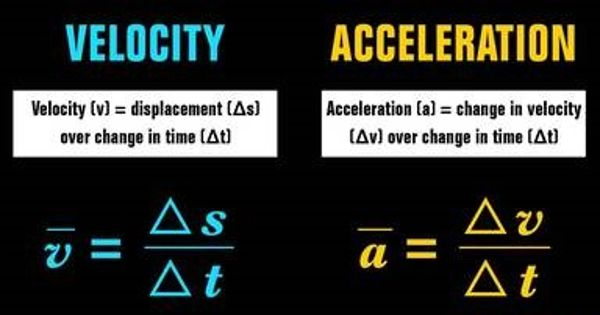Physics

# Difference between Velocity and AccelerationVelocity and acceleration both use speed as a starting point in their measurements. Velocity and acceleration are the two key concepts that are always discussed while studying motion. The amount of motion normalized to time is defined to be the velocity of the object. Velocity can be understood as the speed of a moving body in a particular direction whereas acceleration is any change in the velocity of the object, with respect to time. Acceleration is a vector quantity that is defined as the rate at which an object changes its velocity.

The unit of measurement of velocity is meter per second (m/s) whereas the standard unit of acceleration is meter per second squared (m/s2).

VELOCITY

• Velocity implies the speed of an object, in the given direction. It is an object refers to the speed in a specific direction.
• In physics, velocity is described as a vector measurement as it has both magnitude and direction, wherein the magnitude represents speed and direction show its direction of motion.
• Velocity is nothing but the rate of change of displacement.
• Velocity is a physical quantity which describes the rate at which object moves, along with its direction. It implies the rate of change in position of someone or something, with respect to time, i.e. how fast an object displaces itself over time from one point to another.
• Velocity determines the speed of the moving object along with the direction of motion.
• Velocity is calculated as displacement divided by the time taken in which it takes place.

ACCELERATION

• Acceleration alludes to any change in the velocity of the object with respect to time. It implies any change in the velocity of the object with respect to time.
• A measure of change in velocity, with respect to time, is termed as acceleration. Whenever an object changes its velocity, it is said to be accelerating. It is a vector expression that has both magnitude and direction.
• Acceleration is the rate of change of velocity with respect to time.
• The change in object’s speed and direction are indicated by the component of acceleration, i.e. direction. When the direction of acceleration is parallel to velocity, then it is believed that object is accelerating or its speed is going up.
• Acceleration ascertains the speed of change in velocity of the moving object over a period of time.
• Acceleration can be calculated as: change in velocity divided by the time taken while the change took place.

Information Source: